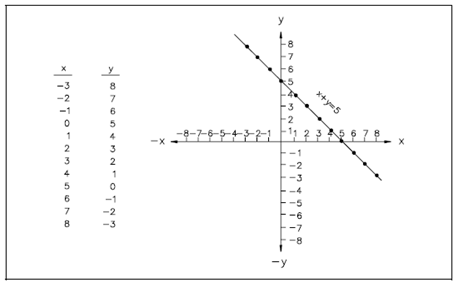## Graphing Equations Assignment Help

Assignment Help: >> Graphing - Graphing Equations

Graphing Equations:

Algebraic equations including two unknowns can readily be display on a graph.  Figure shows a plot of the equation x + y = 5.  The equation is solved for corresponding sets of values of x and y that satisfy the equation.  Every of these points is plotted and the points linked.  The graph of x + y = 5 is a straight line.Figure: Plot of x + y = 5

The x-intercept of a line on a graph is described as the value of the x-coordinate while the y-coordinate is zero.  It is the value of x where the graph intercepts the x-axis.  The y-intercept of a graph is described as the value of the y-coordinate while the x-coordinate is zero. It is the value of y where the graph intercepts the y-axis.#### Assured A++ Grade

Get guaranteed satisfaction & time on delivery in every assignment order you paid with us! We ensure premium quality solution document along with free turntin report!